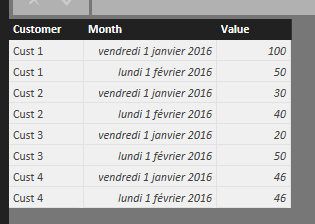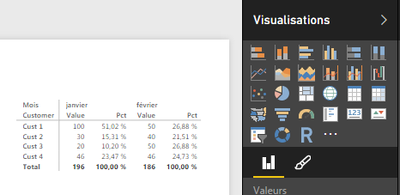cancel
Showing results for
Did you mean:Skilled Sharer

## Monthly Percentage

Hello,

http://community.powerbi.com/t5/Desktop/Monthly-Comparison/m-p/22548
Now I want to add the percentage each value constitutes of the whole month.

I created a simlpe column Percentage = MonthlyValue/SUM(MonthlyValue) but when putting it in my visual (matrix), it calculates the portion of the value over all values providede in all months and not only the month in question.

How can I replicate the attached screenshot in Power BI?Thank you.

1 ACCEPTED SOLUTIONThe difference is, that my [MonthlyValue] is e measure, and yours is the column name without aggregation. Put a SUM() around your "Table13[Monthly Value]" --> SUM( Table13[Monthly Value] ).

The complete formula look like this:

=SUM( Table13[Monthly Value] ) /

CALCULATE(

SUM( Table13[Monthly Value] ),

ALL(Tabelle13[Column1])

)

Does it work now? Is column1 the column with the customer?! Never use Columns without an aggregator (eg. SUM())!

Regards,

Lars

9 REPLIES 9Skilled Sharer

It still did not work as a column even when adding SUM() but it worked as a measure. These two types still get me very confused.

Merci @AnonymousAnonymous
Not applicable

Hi @SabineOussi,

the difference between calculated columns and measures is :

• A Calculated Column runs the calculation on each individual row.  The calculation happens BEFORE aggregating.
• A Calculated Measure runs the calculation on a collection of rows.  Calculations happen AFTER aggregating.Anonymous
Not applicable

Hello,

I'm sorry my previous post was incomplete, I've updated it now 🙂

You're Welcome @SabineOussi and sorry for the delay

SebastienHi @SabineOussi,

try the following formula for the percentages:

Pct = [MonthlyValue] /

CALCULATE(

[MonthlyValue];

ALL(Tabelle1[Customer])

)

Regards,

LarsSkilled Sharer

Sorry but it did not work, wrong values.

Any other approach?Hi @SabineOussi,

did you use your Table-Names and Column Names???... In my case the table name is "Tabelle1"... The formula worked fine in my model.

The main point is the ALL()-function within the CALCULATE().

Regards,

LarsSkilled Sharer

This is what I tried and got.I even tried sum and count after calculate but none was right.The difference is, that my [MonthlyValue] is e measure, and yours is the column name without aggregation. Put a SUM() around your "Table13[Monthly Value]" --> SUM( Table13[Monthly Value] ).

The complete formula look like this:

=SUM( Table13[Monthly Value] ) /

CALCULATE(

SUM( Table13[Monthly Value] ),

ALL(Tabelle13[Column1])

)

Does it work now? Is column1 the column with the customer?! Never use Columns without an aggregator (eg. SUM())!

Regards,

LarsAnonymous
Not applicable

Hello @SabineOussi

The last @LarsSchreiber formula is working pretty well !

Here's the screenshot of what I did :

The model :the formulaAnd the result :Sébastien